C# Delegates and Events Tutorial with Examples

View more Tutorials: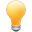Follow us on our fanpages to receive notifications every time there are new articles.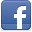Facebook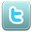Twitter

1- What is Delegate?

In C#, each of function (method or constructor) has a type of function. Let's see the SayHello method as below: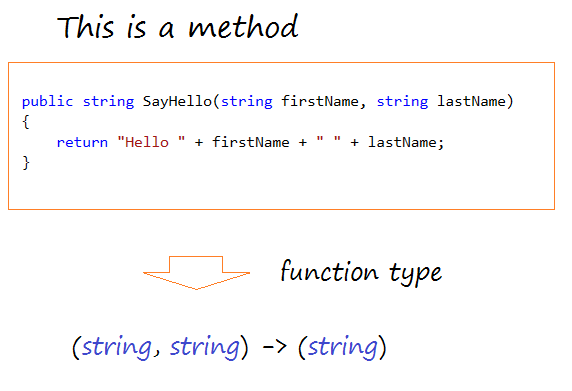View full example:
HelloProgram.cs

using System;
using System.Collections.Generic;
using System.Linq;
using System.Text;

namespace CSharpDelegatesTutorial
{
class HelloProgram
{
// This is the method that has a parameter of type string and returns the string
// Function type: (string) -> (string)
public string SayHello(string name)
{
return "Hello " + name;
}

// This is the method that has 2 parameter and returns the string
// Function type:  (string, string) -> (string)
public string SayHello(string firstName, string lastName)
{
return "Hello " + firstName + " " + lastName;
}

// This is the method that has a parameter and returns nothing.
// Function type: (string) -> ()
public void Silent(string name)
{

}
}

}
The two below methods are in a same function type: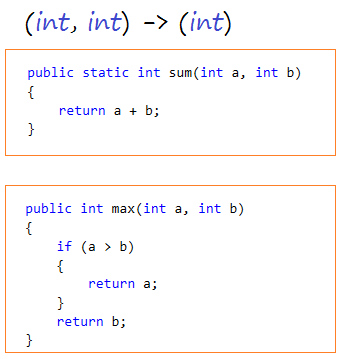C# uses the delegate keyword to define an entity representing for functions (method or constructor) with a same function-type.
Syntax:

// Syntax to define a delegate:
delegate <return_type> <delegate_name> <parameter_list>
Example

// Define a type representing the function type:
// (string,string) -> (string).
private delegate string MyDelegate(string s1, string s2);

2- Delegate examples

MathUtils.cs

using System;
using System.Collections.Generic;
using System.Linq;
using System.Text;

namespace CSharpDelegatesTutorial
{
class MathUtils
{

// (int, int)  -> (int)
public static int sum(int a, int b)
{
return a + b;
}

// (int, int)  -> (int)
public static int minus(int a, int b)
{
return a - b;
}

// (int, int)  -> (int)
public static int multiple(int a, int b)
{
return a * b;
}

}

}
The below example defines a delegate IntIntToInt representing for the functions as (int, int) -> (int).
MyFirstDelegate.cs

using System;
using System.Collections.Generic;
using System.Linq;
using System.Text;

namespace CSharpDelegatesTutorial
{
class MyFirstDelegate
{
// Defining a 'delegate' for the function type:
// (int, int) -> (int)
delegate int IntIntToInt(int a, int b);

public static void Main(string[] args)
{
// Create a delegate object
// Pass a function to it. (Same function type with delegate)
IntIntToInt iiToInt = new IntIntToInt(MathUtils.sum);

// Execute a delegate
// It will call the function (or method) that it represents.
int value = iiToInt(10, 20); // 30

Console.WriteLine("Value = {0}", value);

// Assign other values for delegate
iiToInt = new IntIntToInt(MathUtils.multiple);

value = iiToInt(10, 20); // 200

Console.WriteLine("Value = {0}", value);

}

}

}
Running the example:

Value = 30
Value = 200
You can also create the delegate representing for none static function. Seeing the below example:
HelloProgramTest.cs

using System;
using System.Collections.Generic;
using System.Linq;
using System.Text;

namespace CSharpDelegatesTutorial
{
class HelloProgramTest
{
// Create a Delegate
// Representing the type of function: (string) -> (string).
private delegate string StringStringToString(string s);

public static void Main(string[] args)
{

// Create HelloProgram object.
HelloProgram program = new HelloProgram();

// Create a delegate, represent for sayHello function
StringStringToString ssToS = new StringStringToString(program.SayHello);

// Test
string greeting = ssToS("Tom");

Console.WriteLine(greeting);

}

}

}

3- Function returns a function

In C#, for Delegate you can create a function returning a function (In fact: a function returns a Delegate).
TaxFormulas.cs

using System;
using System.Collections.Generic;
using System.Linq;
using System.Text;

namespace CSharpDelegatesTutorial
{
class TaxFormulas
{
// A delegate representing the type of function: (float) -> (float).
public delegate float TaxFormula(float salary);

// US tax calculation formula (10% of salary).
public static float UsaFormula(float salary)
{
return 10 * salary / 100;
}

// Vietnam tax calculation formula (5% of salary).
public static float VietnamFormula(float salary)
{
return 5 * salary / 100;
}

// Default tax calculation formula (7% of salary).
public static float DefaultFormula(float salary)
{
return 7 * salary / 100;
}

// Returns a function to calculate the tax,
// based on the national code (VN,USA,..)
public static TaxFormula GetSalaryFormula(string countryCode)
{
if (countryCode == "VN")
{
return TaxFormulas.VietnamFormula;
}
else if (countryCode == "USA")
{
return TaxFormulas.UsaFormula;
}
return TaxFormulas.DefaultFormula;
}

}

}
TaxFormulaTest.cs

using System;
using System.Collections.Generic;
using System.Linq;
using System.Text;

namespace CSharpDelegatesTutorial
{
class TaxFormulaTest
{

public static void Main(string[] args)
{
float salary = 1000f;

// The formula for calculating tax in Vietnam.
TaxFormulas.TaxFormula formula = TaxFormulas.GetSalaryFormula("VN");

float tax = formula(salary);

Console.WriteLine("Tax in Vietnam = {0}", tax);

// The formula for calculating tax in Canada.
formula = TaxFormulas.GetSalaryFormula("CA");

tax = formula(salary);

Console.WriteLine("Tax in Canada = {0}", tax);

}
}

}
Running the example:

Tax in Vietname = 50

4- Anonymous methods

From Delegate, you can create an anonymous method, which has no name but body, and is a block with 0 or mores parameters or return type.
AnonymousMethod.cs

using System;
using System.Collections.Generic;
using System.Linq;
using System.Text;

namespace CSharpDelegatesTutorial
{
class AnonymousMethod
{
// A delegate representing the type of function: (float) -> (float).
// Tax calculation based on salary.
public delegate float TaxFormula(float salary);

public static TaxFormula GetTaxFormula(string countryCode)
{
if ("USA" == countryCode)
{
TaxFormula usaFormula = delegate(float salary)
{
return 10 * salary / 100;
};
return usaFormula;
}
else if ("VN" == countryCode)
{
TaxFormula vnFormula = delegate(float salary)
{
return 5 * salary / 100;
};
return vnFormula;
}
return delegate(float salary)
{
return 7 * salary / 100;
};
}

public static void Main(string[] args)
{
string countryCode = "VN";
float salary = 1000;

TaxFormula formula = GetTaxFormula(countryCode);

float tax = formula(salary);

Console.WriteLine("countryCode = {0}, salary = {1} -> tax = {2}"
, countryCode, salary, tax);

}
}

}
Running the example:

countryCode = VN, salary = 1000 -> tax = 50

5- Multicasting of a Delegate

C# allows you to add (+) two Delegates to form a new Delegate. Noting that the Delegates added must be in the same funtiontype, and in the function without return type. When the new Delegate is executed, all sub-Delegates will also be executed. This is called as the Multicasting of delegate.
Greetings.cs

using System;
using System.Collections.Generic;
using System.Linq;
using System.Text;

namespace CSharpDelegatesTutorial
{
class Greetings
{
// Function type:  (String) -> ()
public static void Hello(String name)
{
Console.WriteLine("Hello " + name);
}

// Function type:  (String) -> ()
public static void Bye(string name)
{
Console.WriteLine("Bye " + name);
}

// Function type:  (String) -> ()
public static void Hi(string name)
{
Console.WriteLine("Hi " + name);
}
}

}
MulticastingTest.cs

using System;
using System.Collections.Generic;
using System.Linq;
using System.Text;

namespace CSharpDelegatesTutorial
{
class MulticastingTest
{
// Declare a Delegate.
public delegate void Greeting(string name);

public static void Main(string[] args)
{
// Create delegate objects.
Greeting hello = new Greeting(Greetings.Hello);
Greeting bye = new Greeting(Greetings.Bye);
Greeting hi = new Greeting(Greetings.Hi);

// Create a delegate (sum of two delegate).
Greeting greeting = hello + bye;

// You are also using += operator.
greeting += hi;

// Execute greeting.
greeting("Tom");

}
}

}
Running the example:

Hello Tom
Bye Tom
Hi Tom

6- What is Event?

For C#, Event is a special object of Delegate, which contains methods being executed simultaneously when the event happen. You can add the methods which will be executed on Event of the object raising the event.
Example:
Button class simulates a button, which defines an Event in order to inform that it just been clicked. When the button is clicked, the event will be executed. You need to add the methods requiring the execution for the Event from outside.
Button.cs

using System;
using System.Collections.Generic;
using System.Linq;
using System.Text;

namespace CSharpEventsTutorial
{
class Button
{

private string label;

public delegate void ClickHander(Button source, int x, int y);

// Define an event, it has not been assigned value.
// Its value is assigned from outside.
public event ClickHander OnButtonClick;

public Button(string label)
{
this.label = label;
}

// Simulate this Button is clicked.
// Determine the position x, y the user click.
public void Clicked()
{
Random random = new Random();

// A random number between 1 -> 100
int x = random.Next(1, 100);

// A random number between 1 -> 20
int y = random.Next(1, 20);

if (OnButtonClick != null)
{
// Call event handlers.
OnButtonClick(this, x, y);
}

}
}

}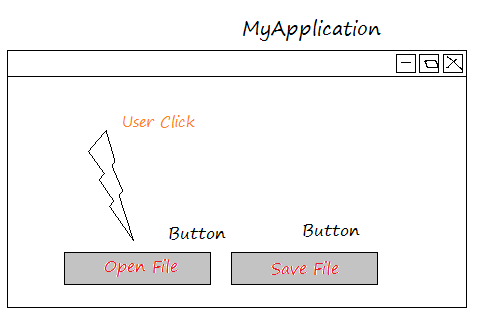Class MyApplication simulates a application with 2 button,  "Open File" and "Save File".

You need to write the method to do something when user clicks on "Open File", and add this method to the OnButtonClick event of openButton button.

You need to write the method to do something when user clikcks on "Save File", and add this method to the OnButtonClick event of saveButton button.
MyApplication.cs

using System;
using System.Collections.Generic;
using System.Linq;
using System.Text;

namespace CSharpEventsTutorial
{
class MyApplication
{
private Button openButton;
private Button saveButton;
private string fileName;

// Simulating an application with the buttons.
public MyApplication()
{
// Add a button to interface.
this.openButton = new Button("Open File");

// Add a button to interface
this.saveButton = new Button("Save File");

// Add method to event of button. ('Open Button')
// (Multicasting feature of Delegate)
this.openButton.OnButtonClick += this.OpenButtonClicked;

// Add method to event of button ('Save Button').
// (Multicasting feature of Delegate)
this.saveButton.OnButtonClick += this.SaveButtonClicked;
}

private void OpenButtonClicked(Button source, int x, int y)
{
// Simulate opens a window to select File to open.
Console.WriteLine("Open Dialog to Select a file");
//
this.fileName = "File" + x + "_" + y+".txt";
Console.WriteLine("Openning file: "+ this.fileName);
}

private void SaveButtonClicked(Button source, int x, int y)
{
if(this.fileName== null)  {
Console.WriteLine("No file to save!");
return;
}
// Save File
Console.WriteLine("Saved file: " + this.fileName);
}

public static void Main(string[] args)
{

// Open app
MyApplication myApp = new MyApplication();

Console.WriteLine("User Click on Open Button ....");

// Click openButton
myApp.openButton.Clicked();

Console.WriteLine("\n\n");
Console.WriteLine("User Click on Save Button ....");

// Click saveButton.
myApp.saveButton.Clicked();

}
}

}
Running the example:

User Click on Open Button ....
Open Dialog to Select a file
Openning file: File75_4.txt

User Click on Save Button ....
Saved file: File75_4.txt

View more Tutorials:

Maybe you are interested

These are online courses outside the o7planning website that we introduced, which may include free or discounted courses.

•Learn and Understand Interfaces in C#
•C# Basics - Learn to Code the Right Way
•Design Patterns in C# and .NET
•Complete C++, C# and C programming tools for complete NOVICE
•A beginners guide to C# Scripting for Unity Game Development
•Learn to code by creating 14 projects with JavaScript and C#
•Intro to C# Programming and Scripting for Games in Unity
•Xamarin Android - A Master Guide to App Development in C#
•Learn C# From Scratch with Real Applications
•C# Design patterns
•Starting 2D Game Development in Unity with C#
•The complete algorithms course in C# and .Net
•Complete C# Unity Developer 2D: Learn to Code Making Games
•Naked C#: A Beginner's Guide to Coding
•Intro to C# Programming and Scripting for Games in Unity
•Learn ASP NET with Bootstrap,Entity Framework,JavaScript,C#
•Internet of things development for C# programmers
•C# Basics - Learn Coding & Programming for Beginners
•An Introduction to C# for the Rest of Us
•C# Loops for Beginners
•C# Beginner To Professional Software Developer
•What's New in C#7 and C# 8
•ASP NET Core 3 (ASP.NET 5),MVC,C#,Angular & EF Crash Course
•C# Programming from Zero to Hero : The Fundamentals
•Unity C# Editor Scripting Masterclass